Theory and Modern Applications

# Non-Archimedean Hyers-Ulam-Rassias stability of m-variable functional equation

## Abstract

The main goal of this paper is to study the Hyers-Ulam-Rassias stability of the following Euler-Lagrange type additive functional equation:

$\sum _{j=1}^{m}f\left(-{r}_{j}{x}_{j}+\sum _{1\le i\le m,i\ne j}{r}_{i}{x}_{i}\right)+2\sum _{i=1}^{m}{r}_{i}f\left({x}_{i}\right)=mf\left(\sum _{i=1}^{m}{r}_{i}{x}_{i}\right),$

where ${r}_{1},\dots ,{r}_{m}\in \mathbb{R}$, ${\sum }_{i=k}^{m}{r}_{k}\ne 0$, and ${r}_{i},{r}_{j}\ne 0$ for some $1\le i, in non-Archimedean Banach spaces.

MSC:39B22, 39B52, 46S10.

## 1 Introduction and preliminaries

A valuation is a function $|\cdot |$ from a field $\mathbb{K}$ into $\left[0,\mathrm{\infty }\right)$ such that 0 is the unique element having the 0 valuation, $|rs|=|r||s|$ and the triangle inequality is replaced by $|r+s|\le max\left\{|r|,|s|\right\}$.

The field $\mathbb{K}$ is called a valued field if $\mathbb{K}$ carries a valuation. The usual absolute values of $\mathbb{R}$ and $\mathbb{C}$ are the examples of valuations.

Let us consider the valuation which satisfies a stronger condition than the triangle inequality. If the triangle inequality is replaced by $|r+s|\le max\left\{|r|,|s|\right\}$ for all $r,s\in \mathbb{K}$, then the function $|\cdot |$ is called a non-Archimedean valuation and the field is called a non-Archimedean field. Clearly, $|1|=|-1|=1$ and $|n|\le 1$ for all integers $n\ge 1$. A trivial example of a non-Archimedean valuation is the function $|\cdot |$ taking everything except for 0 into 1 and $|0|=0$.

Definition 1.1 Let X be a vector space over a field $\mathbb{K}$ with a non-Archimedean valuation $|\cdot |$. A function $\parallel \cdot \parallel :X\to \left[0,\mathrm{\infty }\right)$ is called a non-Archimedean norm if the following conditions hold:

1. (a)

$\parallel x\parallel =0$ if and only if $x=0$ for all $x\in X$;

2. (b)

$\parallel rx\parallel =|r|\parallel x\parallel$ for all $r\in \mathbb{K}$ and $x\in X$;

3. (c)

the strong triangle inequality holds:

$\parallel x+y\parallel \le max\left\{\parallel x\parallel ,\parallel y\parallel \right\}$

for all $x,y\in X$.

Then $\left(X,\parallel \cdot \parallel \right)$ is called a non-Archimedean normed space.

Definition 1.2 Let $\left\{{x}_{n}\right\}$ be a sequence in a non-Archimedean normed space X.

1. (a)

A sequence ${\left\{{x}_{n}\right\}}_{n=1}^{\mathrm{\infty }}$ in a non-Archimedean space is a Cauchy sequence iff the sequence ${\left\{{x}_{n+1}-{x}_{n}\right\}}_{n=1}^{\mathrm{\infty }}$ converges to zero;

2. (b)

The sequence $\left\{{x}_{n}\right\}$ is said to be convergent if, for any $\epsilon >0$, there is a positive integer N and $x\in X$ such that $\parallel {x}_{n}-x\parallel \le \epsilon$, for all $n\ge N$. Then the point $x\in X$ is called the limit of the sequence $\left\{{x}_{n}\right\}$, which is denoted by ${lim}_{n\to \mathrm{\infty }}{x}_{n}=x$;

3. (c)

If every Cauchy sequence in X converges, then the non-Archimedean normed space X is called a non-Archimedean Banach space.

Example 1.1 Fix a prime number p. For any nonzero rational number x, there exists a unique integer ${n}_{x}\in \mathbb{Z}$ such that $x=\frac{a}{b}{p}^{{n}_{x}}$, where a and b are integers not divisible by p. Then ${|x|}_{p}:={p}^{-{n}_{x}}$ defines a non-Archimedean norm on $\mathbb{Q}$. The completion of $\mathbb{Q}$ with respect to the metric $d\left(x,y\right)={|x-y|}_{p}$ is denoted by ${\mathbb{Q}}_{p}$ which is called the p-adic number field. In fact, ${\mathbb{Q}}_{p}$ is the set of all formal series $x={\sum }_{k\ge {n}_{x}}^{\mathrm{\infty }}{a}_{k}{p}^{k}$ where $|{a}_{k}|\le p-1$ are integers. The addition and multiplication between any two elements of ${\mathbb{Q}}_{p}$ are defined naturally. The norm ${|{\sum }_{k\ge {n}_{x}}^{\mathrm{\infty }}{a}_{k}{p}^{k}|}_{p}={p}^{-{n}_{x}}$ is a non-Archimedean norm on ${\mathbb{Q}}_{p}$ and it makes ${\mathbb{Q}}_{p}$ a locally compact field.

Theorem 1.1 Let$\left(X,d\right)$be a complete generalized metric space and$J:X\to X$be a strictly contractive mapping with Lipschitz constant$L<1$. Then, for all$x\in X$, either$d\left({J}^{n}x,{J}^{n+1}x\right)=\mathrm{\infty }$for all nonnegative integers n or there exists a positive integer${n}_{0}$such that:

1. (a)

$d\left({J}^{n}x,{J}^{n+1}x\right)<\mathrm{\infty }$for all${n}_{0}\ge {n}_{0}$;

2. (b)

the sequence$\left\{{J}^{n}x\right\}$converges to a fixed point${y}^{\ast }$of J;

3. (c)

${y}^{\ast }$is the unique fixed point of J in the set$Y=\left\{y\in X:d\left({J}^{{n}_{0}}x,y\right)<\mathrm{\infty }\right\}$;

4. (d)

$d\left(y,{y}^{\ast }\right)\le \frac{1}{1-L}d\left(y,Jy\right)$for all$y\in Y$.

In this paper, we prove the generalized Hyers-Ulam stability of the following functional equation:

$\sum _{j=1}^{m}f\left(-{r}_{j}{x}_{j}+\sum _{1\le i\le m,i\ne j}{r}_{i}{x}_{i}\right)+2\sum _{i=1}^{m}{r}_{i}f\left({x}_{i}\right)=mf\left(\sum _{i=1}^{m}{r}_{i}{x}_{i}\right),$
(1.1)

where ${r}_{1},\dots ,{r}_{m}\in \mathbb{R}$, ${\sum }_{k=1}^{m}{r}_{k}\ne 0$, and ${r}_{i},{r}_{j}\ne 0$ for some $1\le i, in non-Archimedean Banach spaces. A classical question in the theory of functional equations is the following: ‘When is it true that a function which approximately satisfies a functional equation D must be close to an exact solution of D?’.

If the problem accepts a solution, we say that the equation D is stable. The first stability problem concerning group homomorphisms was raised by Ulam  in 1940.

In the next year D. H. Hyres , gave a positive answer to the above question for additive groups under the assumption that the groups are Banach spaces. In 1978, Th. M. Rassias  proved a generalization of Hyres’ theorem for additive mappings.

The result of Th. M. Rassias has influenced the development of what is now called the Hyers-Ulam-Rassias stability theory for functional equations. In 1994, a generalization of Rassias’ theorem was obtained by Gǎvruta  by replacing the bound $ϵ\left({\parallel x\parallel }^{p}+{\parallel y\parallel }^{p}\right)$ by a general control function $\phi \left(x,y\right)$.

The stability problems of several functional equations have been extensively investigated by a number of authors and there are many interesting results concerning this problem (see ).

## 2 Non-Archimedean stability of the functional equation (1.1): a fixed point approach

In this section, using a fixed point alternative approach, we prove the generalized Hyers-Ulam stability of the functional equation (1.1) in non-Archimedean normed spaces. Throughout this section, let X be a non-Archimedean normed space and Y be a non-Archimedean Banach space. Also $|2|\ne 1$.

Lemma 2.1 Let$\mathcal{X}$and$\mathcal{Y}$be linear spaces and let${r}_{1},\dots ,{r}_{n}$be real numbers with${\sum }_{k=1}^{n}{r}_{k}\ne 0$and${r}_{i},{r}_{j}\ne 0$for some$1\le i. Assume that a mapping$f:\mathcal{X}\to \mathcal{Y}$satisfies the functional equation (1.1) for all${x}_{1},\dots ,{x}_{n}\in \mathcal{X}$. Then the mapping f is Cauchy additive. Moreover, $f\left({r}_{k}x\right)={r}_{k}f\left(x\right)$for all$x\in \mathcal{X}$and all$1\le k\le n$.

Proof Since ${\sum }_{k=1}^{n}{r}_{k}\ne 0$, putting ${x}_{1}=\cdots ={x}_{n}=0$ in (1.1), we get $f\left(0\right)=0$. Without loss of generality, we may assume that ${r}_{1},{r}_{2}\ne 0$. Letting ${x}_{3}=\cdots ={x}_{n}=0$ in (1.1), we get

$f\left(-{r}_{1}{x}_{1}+{r}_{2}{x}_{2}\right)+f\left({r}_{1}{x}_{1}-{r}_{2}{x}_{2}\right)+2{r}_{1}f\left({x}_{1}\right)+2{r}_{2}f\left({x}_{2}\right)=2f\left({r}_{1}{x}_{1}+{r}_{2}{x}_{2}\right)$
(2.1)

for all ${x}_{1},{x}_{2}\in \mathcal{X}$. Letting ${x}_{2}=0$ in (2.1), we get

$2{r}_{1}f\left({x}_{1}\right)=f\left({r}_{1}{x}_{1}\right)-f\left(-{r}_{1}{x}_{1}\right)$
(2.2)

for all ${x}_{1}\in \mathcal{X}$. Similarly, by putting ${x}_{1}=0$ in (2.1), we get

$2{r}_{2}f\left({x}_{2}\right)=f\left({r}_{2}{x}_{2}\right)-f\left(-{r}_{2}{x}_{2}\right)$
(2.3)

for all ${x}_{1}\in \mathcal{X}$. It follows from (2.1), (2.2) and (2.3) that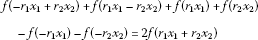(2.4)

for all ${x}_{1},{x}_{2}\in \mathcal{X}$. Replacing ${x}_{1}$ and ${x}_{2}$ by $\frac{x}{{r}_{1}}$ and $\frac{y}{{r}_{2}}$ in (2.4), we get

$f\left(-x+y\right)+f\left(x-y\right)+f\left(x\right)+f\left(y\right)-f\left(-x\right)-f\left(-y\right)=2f\left(x+y\right)$
(2.5)

for all $x,y\in \mathcal{X}$. Letting $y=-x$ in (2.5), we get that $f\left(-2x\right)+f\left(2x\right)=0$ for all $x\in \mathcal{X}$. So the mapping L is odd. Therefore, it follows from (2.5) that the mapping f is additive. Moreover, let $x\in \mathcal{X}$ and $1\le k\le n$. Setting ${x}_{k}=x$ and ${x}_{l}=0$ for all $1\le l\le n$, $l\ne k$, in (1.1) and using the oddness of f, we get that $f\left({r}_{k}x\right)={r}_{k}f\left(x\right)$. □

Using the same method as in the proof of Lemma 2.1, we have an alternative result of Lemma 2.1 when ${\sum }_{k=1}^{n}{r}_{k}=0$.

Lemma 2.2 Let$\mathcal{X}$and$\mathcal{Y}$be linear spaces and let${r}_{1},\dots ,{r}_{n}$be real numbers with${r}_{i},{r}_{j}\ne 0$for some$1\le i. Assume that a mapping$f:\mathcal{X}\to \mathcal{Y}$with$f\left(0\right)=0$satisfying the functional equation (1.1) for all${x}_{1},\dots ,{x}_{n}\in \mathcal{X}$. Then the mapping f is Cauchy additive. Moreover, $f\left({r}_{k}x\right)={r}_{k}f\left(x\right)$for all$x\in \mathcal{X}$and all$1\le k\le n$.

Remark 2.1 Throughout this paper, ${r}_{1},\dots ,{r}_{m}$ will be real numbers such that ${r}_{i},{r}_{j}\ne 0$ for fixed $1\le i and ${\phi }_{i,j}\left(x,y\right):=\phi \left(0,\dots ,0,\underset{i\mathrm{th}}{\underset{⏟}{x}},0,\dots ,0,\underset{j\mathrm{th}}{\underset{⏟}{y}},0,\dots ,0\right)$ for all $x,y\in X$ and all $1\le i.

Theorem 2.1 Let $\phi :{X}^{m}\to \left[0,\mathrm{\infty }\right)$ be a function such that there exists an $L<1$ with

$\phi \left(\frac{{x}_{1}}{2},\dots ,\frac{{x}_{m}}{2}\right)\le \frac{L\phi \left({x}_{1},\dots ,{x}_{m}\right)}{|2|}$
(2.6)

for all${x}_{1},\dots ,{x}_{m}\in X$. Let$f:X\to Y$be a mapping with$f\left(0\right)=0$satisfying the following inequality: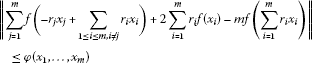(2.7)

for all${x}_{1},\dots ,{x}_{m}\in X$. Then there is a unique Euler-Lagrange type additive mapping$\mathit{EL}:X\to Y$such that

$\begin{array}{rcl}\parallel f\left(x\right)-\mathit{EL}\left(x\right)\parallel & \le & \frac{L}{|2|-|2|L}max\left\{max\left\{{\phi }_{i,j}\left(\frac{x}{2{r}_{i}},-\frac{x}{2{r}_{j}}\right),{\phi }_{i,j}\left(\frac{x}{2{r}_{i}},0\right),{\phi }_{i,j}\left(0,-\frac{x}{2{r}_{j}}\right)\right\},\\ \frac{1}{|2|}max\left\{{\phi }_{i,j}\left(\frac{x}{{r}_{i}},\frac{x}{{r}_{j}}\right),{\phi }_{i,j}\left(\frac{x}{{r}_{i}},0\right),{\phi }_{i,j}\left(0,\frac{x}{{r}_{j}}\right)\right\}\right\}\end{array}$
(2.8)

for all$x\in X$.

Proof For each $1\le k\le m$ with $k\ne i,j$, let ${x}_{k}=0$ in (2.7). Then we get the following inequality: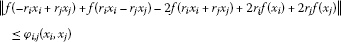(2.9)

for all ${x}_{i},{x}_{j}\in X$. Letting ${x}_{i}=0$ in (2.9), we get

$\parallel f\left(-{r}_{j}{x}_{j}\right)-f\left({r}_{j}{x}_{j}\right)+2{r}_{j}f\left({x}_{j}\right)\parallel \le {\phi }_{i,j}\left(0,{x}_{j}\right)$
(2.10)

for all ${x}_{j}\in X$. Similarly, letting ${x}_{j}=0$ in (2.9), we get

$\parallel f\left(-{r}_{i}{x}_{i}\right)-f\left({r}_{i}{x}_{i}\right)+2{r}_{i}f\left({x}_{i}\right)\parallel \le {\phi }_{i,j}\left({x}_{i},0\right)$
(2.11)

for all ${x}_{i}\in X$. It follows from (2.9), (2.10) and (2.11) that for all ${x}_{i},{x}_{j}\in X$(2.12)

Replacing ${x}_{i}$ and ${x}_{j}$ by $\frac{x}{{r}_{i}}$ and $\frac{y}{{r}_{j}}$ in (2.12), we get that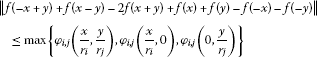(2.13)

for all $x,y\in X$. Putting $y=x$ in (2.13), we get

$\parallel f\left(x\right)-f\left(-x\right)-f\left(2x\right)\parallel \le \frac{1}{|2|}max\left\{{\phi }_{i,j}\left(\frac{x}{{r}_{i}},\frac{x}{{r}_{j}}\right),{\phi }_{i,j}\left(\frac{x}{{r}_{i}},0\right),{\phi }_{i,j}\left(0,\frac{x}{{r}_{j}}\right)\right\}$
(2.14)

for all $x\in X$. Replacing x and y by $\frac{x}{2}$ and $-\frac{x}{2}$ in (2.13) respectively, we get

$\parallel f\left(x\right)+f\left(-x\right)\parallel \le max\left\{{\phi }_{i,j}\left(\frac{x}{2{r}_{i}},-\frac{x}{2{r}_{j}}\right),{\phi }_{i,j}\left(\frac{x}{2{r}_{i}},0\right),{\phi }_{i,j}\left(0,-\frac{x}{2{r}_{j}}\right)\right\}$
(2.15)

for all $x\in X$. It follows from (2.14) and (2.15) that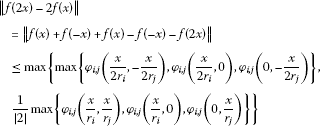(2.16)

for all $x\in X$. Replacing x by $\frac{x}{2}$ in (2.16), we obtain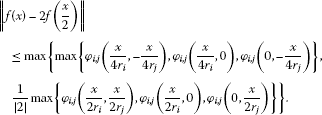(2.17)

Consider the set $S:=\left\{g:X\to Y;g\left(0\right)=0\right\}$ and the generalized metric d in S defined by

$\begin{array}{rcl}d\left(f,g\right)& =& \underset{\mu \in {\mathbb{R}}^{+}}{inf}\left\{\parallel g\left(x\right)-h\left(x\right)\parallel \le \mu max\left\{max\left\{{\phi }_{i,j}\left(\frac{x}{2{r}_{i}},-\frac{x}{2{r}_{j}}\right),\\ {\phi }_{i,j}\left(\frac{x}{2{r}_{i}},0\right),{\phi }_{i,j}\left(0,-\frac{x}{2{r}_{j}}\right)\right\},\\ \frac{1}{|2|}max\left\{{\phi }_{i,j}\left(\frac{x}{{r}_{i}},\frac{x}{{r}_{j}}\right),{\phi }_{i,j}\left(\frac{x}{{r}_{i}},0\right),{\phi }_{i,j}\left(0,\frac{x}{{r}_{j}}\right)\right\}\right\},\mathrm{\forall }x\in X\right\},\end{array}$

where $inf\mathrm{\varnothing }=+\mathrm{\infty }$. It is easy to show that $\left(S,d\right)$ is complete (see , Lemma 2.1). Now, we consider a linear mapping $J:S\to S$ such that

$Jh\left(x\right):=2h\left(\frac{x}{2}\right)$

for all $x\in X$. Let $g,h\in S$ be such that $d\left(g,h\right)=ϵ$. Then

$\begin{array}{rcl}\parallel g\left(x\right)-h\left(x\right)\parallel & \le & ϵmax\left\{max\left\{{\phi }_{i,j}\left(\frac{x}{2{r}_{i}},-\frac{x}{2{r}_{j}}\right),{\phi }_{i,j}\left(\frac{x}{2{r}_{i}},0\right),{\phi }_{i,j}\left(0,-\frac{x}{2{r}_{j}}\right)\right\},\\ \frac{1}{|2|}max\left\{{\phi }_{i,j}\left(\frac{x}{{r}_{i}},\frac{x}{{r}_{j}}\right),{\phi }_{i,j}\left(\frac{x}{{r}_{i}},0\right),{\phi }_{i,j}\left(0,\frac{x}{{r}_{j}}\right)\right\}\right\}\end{array}$

for all $x\in X$, and so

$\begin{array}{rcl}\parallel Jg\left(x\right)-Jh\left(x\right)\parallel & =& \parallel 2g\left(\frac{x}{2}\right)-2h\left(\frac{x}{2}\right)\parallel \\ \le & |2|ϵmax\left\{max\left\{{\phi }_{i,j}\left(\frac{x}{4{r}_{i}},-\frac{x}{4{r}_{j}}\right),{\phi }_{i,j}\left(\frac{x}{4{r}_{i}},0\right),{\phi }_{i,j}\left(0,-\frac{x}{4{r}_{j}}\right)\right\},\\ \frac{1}{|2|}max\left\{{\phi }_{i,j}\left(\frac{x}{2{r}_{i}},\frac{x}{2{r}_{j}}\right),{\phi }_{i,j}\left(\frac{x}{2{r}_{i}},0\right),{\phi }_{i,j}\left(0,\frac{x}{2{r}_{j}}\right)\right\}\right\}\\ \le & |2|\frac{Lϵ}{|2|}max\left\{max\left\{{\phi }_{i,j}\left(\frac{x}{2{r}_{i}},-\frac{x}{2{r}_{j}}\right),{\phi }_{i,j}\left(\frac{x}{2{r}_{i}},0\right),{\phi }_{i,j}\left(0,-\frac{x}{2{r}_{j}}\right)\right\},\\ \frac{1}{|2|}max\left\{{\phi }_{i,j}\left(\frac{x}{{r}_{i}},\frac{x}{{r}_{j}}\right),{\phi }_{i,j}\left(\frac{x}{{r}_{i}},0\right),{\phi }_{i,j}\left(0,\frac{x}{{r}_{j}}\right)\right\}\right\}\end{array}$

for all $x\in X$. Thus $d\left(g,h\right)=ϵ$ implies that $d\left(Jg,Jh\right)\le Lϵ$. This means that

$d\left(Jg,Jh\right)\le Ld\left(g,h\right)$

for all $g,h\in S$. It follows from (2.17) that

$d\left(f,Jf\right)\le \frac{L}{|2|}.$

By Theorem 1.1, there exists a mapping $\mathit{EL}:X\to Y$ satisfying the following:

1. (1)

EL is a fixed point of J, that is,

$\mathit{EL}\left(\frac{x}{2}\right)=\frac{1}{2}\mathit{EL}\left(x\right)$
(2.18)

for all $x\in X$. The mapping EL is a unique fixed point of J in the set

$\mathrm{\Omega }=\left\{h\in S:d\left(g,h\right)<\mathrm{\infty }\right\}.$

This implies that EL is a unique mapping satisfying (2.18) such that there exists $\mu \in \left(0,\mathrm{\infty }\right)$ satisfying

$\begin{array}{rcl}\parallel f\left(x\right)-\mathit{EL}\left(x\right)\parallel & \le & \mu max\left\{max\left\{{\phi }_{i,j}\left(\frac{x}{2{r}_{i}},-\frac{x}{2{r}_{j}}\right),{\phi }_{i,j}\left(\frac{x}{2{r}_{i}},0\right),{\phi }_{i,j}\left(0,-\frac{x}{2{r}_{j}}\right)\right\},\\ \frac{1}{|2|}max\left\{{\phi }_{i,j}\left(\frac{x}{{r}_{i}},\frac{x}{{r}_{j}}\right),{\phi }_{i,j}\left(\frac{x}{{r}_{i}},0\right),{\phi }_{i,j}\left(0,\frac{x}{{r}_{j}}\right)\right\}\right\}\end{array}$
(2.19)

for all $x\in X$.

1. (2)

$d\left({J}^{n}f,\mathit{EL}\right)\to 0$ as $n\to \mathrm{\infty }$. This implies the equality

$\underset{n\to \mathrm{\infty }}{lim}{2}^{n}f\left(\frac{x}{{2}^{n}}\right)=\mathit{EL}\left(x\right)$

for all $x\in X$.

1. (3)

$d\left(f,\mathit{EL}\right)\le \frac{d\left(f,Jf\right)}{1-L}$ with $f\in \mathrm{\Omega }$, which implies the inequality

$d\left(f,\mathit{EL}\right)\le \frac{L}{|2|-|2|L}.$

This implies that the inequality (2.8) holds.

By (2.6) and (2.7), we obtain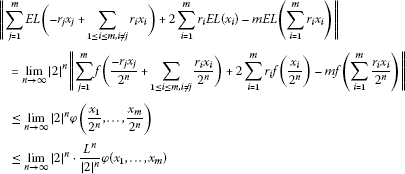for all ${x}_{1},\dots ,{x}_{m}\in X$ and $n\in \mathbb{N}$. So EL satisfies (1.1). Thus, the mapping $\mathit{EL}:X\to Y$ is Euler-Lagrange type additive, as desired. □

Corollary 2.1 Let$\theta \ge 0$and r be a real number with$0. Let$f:X\to Y$be a mapping with$f\left(0\right)=0$satisfying the inequality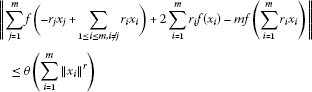(2.20)

for all${x}_{1},\dots ,x\in X$. Then, the limit$\mathit{EL}\left(x\right)={lim}_{n\to \mathrm{\infty }}{2}^{n}f\left(\frac{x}{{2}^{n}}\right)$exists for all$x\in X$and$\mathit{EL}:X\to Y$is a unique Euler-Lagrange additive mapping such that

$\begin{array}{rcl}\parallel f\left(x\right)-\mathit{EL}\left(x\right)\parallel & \le & \frac{|2|}{{|2|}^{r+1}-{|2|}^{2}}max\left\{max\left\{\frac{{|2|}^{r}\theta {\parallel x\parallel }^{r}\left({|{r}_{i}|}^{r}+{|{r}_{j}|}^{r}\right)}{{|4|}^{r}{|{r}_{i}{r}_{j}|}^{r}},\frac{\theta {\parallel x\parallel }^{r}}{{|2|}^{r}{|{r}_{i}|}^{r}},\frac{\theta {\parallel x\parallel }^{r}}{{|2|}^{r}{|{r}_{j}|}^{r}}\right\},\\ \frac{1}{|2|}max\left\{\frac{\theta {\parallel x\parallel }^{r}\left({|{r}_{i}|}^{r}+{|{r}_{j}|}^{r}\right)}{{|{r}_{i}{r}_{j}|}^{r}},\frac{\theta {\parallel x\parallel }^{r}}{{|{r}_{i}|}^{r}},\frac{\theta {\parallel x\parallel }^{r}}{{|{r}_{j}|}^{r}}\right\}\right\}\\ \le & \frac{\theta {\parallel x\parallel }^{r}\left({|{r}_{i}|}^{r}+{|{r}_{j}|}^{r}\right)}{{|{r}_{i}{r}_{j}|}^{r}\left({|2|}^{r+1}-{|2|}^{2}\right)}\end{array}$

for all$x\in X$.

Proof The proof follows from Theorem 2.1 by taking $\phi \left({x}_{1},\dots ,{x}_{m}\right)=\theta \left({\sum }_{i=1}^{m}{\parallel {x}_{i}\parallel }^{r}\right)$ for all ${x}_{1},\dots ,{x}_{m}\in X$. In fact, if we choose $L={|2|}^{1-r}$, then we get the desired result. □

Theorem 2.2 Let $\phi :{X}^{m}\to \left[0,\mathrm{\infty }\right)$ be a function such that there exists an $L<1$ with

$\phi \left({x}_{1},\dots ,{x}_{m}\right)\le |2|L\phi \left(\frac{{x}_{1}}{2},\dots ,\frac{{x}_{m}}{2}\right)$
(2.21)

for all${x}_{1},\dots ,{x}_{m}\in X$. Let$f:X\to Y$be a mapping with$f\left(0\right)=0$satisfying the inequality (2.7). Then, there is a unique Euler-Lagrange additive mapping$\mathit{EL}:X\to Y$such that

$\begin{array}{rcl}\parallel f\left(x\right)-\mathit{EL}\left(x\right)\parallel & \le & \frac{1}{|2|-|2|L}max\left\{max\left\{{\phi }_{i,j}\left(\frac{x}{2{r}_{i}},-\frac{x}{2{r}_{j}}\right),{\phi }_{i,j}\left(\frac{x}{2{r}_{i}},0\right),{\phi }_{i,j}\left(0,-\frac{x}{2{r}_{j}}\right)\right\},\\ \frac{1}{|2|}max\left\{{\phi }_{i,j}\left(\frac{x}{{r}_{i}},\frac{x}{{r}_{j}}\right),{\phi }_{i,j}\left(\frac{x}{{r}_{i}},0\right),{\phi }_{i,j}\left(0,\frac{x}{{r}_{j}}\right)\right\}\right\}.\end{array}$
(2.22)

Proof By (2.16), we have

$\begin{array}{rcl}\parallel \frac{f\left(2x\right)}{2}-f\left(x\right)\parallel & \le & \frac{1}{|2|}max\left\{max\left\{{\phi }_{i,j}\left(\frac{x}{2{r}_{i}},-\frac{x}{2{r}_{j}}\right),{\phi }_{i,j}\left(\frac{x}{2{r}_{i}},0\right),{\phi }_{i,j}\left(0,-\frac{x}{2{r}_{j}}\right)\right\},\\ \frac{1}{|2|}max\left\{{\phi }_{i,j}\left(\frac{x}{{r}_{i}},\frac{x}{{r}_{j}}\right),{\phi }_{i,j}\left(\frac{x}{{r}_{i}},0\right),{\phi }_{i,j}\left(0,\frac{x}{{r}_{j}}\right)\right\}\right\}\end{array}$
(2.23)

for all $x\in X$ . Let $\left(S,d\right)$ be the generalized metric space defined in the proof of Theorem 2.1. Now, we consider a linear mapping $J:S\to S$ such that

$Jh\left(x\right):=\frac{1}{2}h\left(2x\right)$

for all $x\in X$. Let $g,h\in S$ be such that $d\left(g,h\right)=ϵ$. Then

$\begin{array}{rcl}\parallel g\left(x\right)-h\left(x\right)\parallel & \le & ϵmax\left\{max\left\{{\phi }_{i,j}\left(\frac{x}{2{r}_{i}},-\frac{x}{2{r}_{j}}\right),{\phi }_{i,j}\left(\frac{x}{2{r}_{i}},0\right),{\phi }_{i,j}\left(0,-\frac{x}{2{r}_{j}}\right)\right\},\\ \frac{1}{|2|}max\left\{{\phi }_{i,j}\left(\frac{x}{{r}_{i}},\frac{x}{{r}_{j}}\right),{\phi }_{i,j}\left(\frac{x}{{r}_{i}},0\right),{\phi }_{i,j}\left(0,\frac{x}{{r}_{j}}\right)\right\}\right\}\end{array}$

for all $x\in X$, and so

$\begin{array}{rcl}\parallel Jg\left(x\right)-Jh\left(x\right)\parallel & =& \parallel \frac{g\left(2x\right)}{2}-\frac{h\left(2x\right)}{2}\parallel \\ \le & \frac{1}{|2|}ϵmax\left\{max\left\{{\phi }_{i,j}\left(\frac{x}{{r}_{i}},-\frac{x}{{r}_{j}}\right),{\phi }_{i,j}\left(\frac{x}{{r}_{i}},0\right),{\phi }_{i,j}\left(0,-\frac{x}{{r}_{j}}\right)\right\},\\ \frac{1}{|2|}max\left\{{\phi }_{i,j}\left(\frac{2x}{{r}_{i}},\frac{2x}{{r}_{j}}\right),{\phi }_{i,j}\left(\frac{2x}{{r}_{i}},0\right),{\phi }_{i,j}\left(0,\frac{2x}{{r}_{j}}\right)\right\}\right\}\\ \le & |2|L\frac{ϵ}{|2|}max\left\{max\left\{{\phi }_{i,j}\left(\frac{x}{2{r}_{i}},-\frac{x}{2{r}_{j}}\right),{\phi }_{i,j}\left(\frac{x}{2{r}_{i}},0\right),{\phi }_{i,j}\left(0,-\frac{x}{2{r}_{j}}\right)\right\},\\ \frac{1}{|2|}max\left\{{\phi }_{i,j}\left(\frac{x}{{r}_{i}},\frac{x}{{r}_{j}}\right),{\phi }_{i,j}\left(\frac{x}{{r}_{i}},0\right),{\phi }_{i,j}\left(0,\frac{x}{{r}_{j}}\right)\right\}\right\}\end{array}$

for all $x\in X$ . Thus $d\left(g,h\right)=ϵ$ implies that $d\left(Jg,Jh\right)\le Lϵ$. This means that

$d\left(Jg,Jh\right)\le Ld\left(g,h\right)$

for all $g,h\in S$. It follows from (2.23) that

$d\left(f,Jf\right)\le \frac{1}{|2|}.$

By Theorem 1.1, there exists a mapping $\mathit{EL}:X\to Y$ satisfying the following:

1. (1)

EL is a fixed point of J, that is,

$\mathit{EL}\left(2x\right)=2\mathit{EL}\left(x\right)$
(2.24)

for all $x\in X$. The mapping EL is a unique fixed point of J in the set

$\mathrm{\Omega }=\left\{h\in S:d\left(g,h\right)<\mathrm{\infty }\right\}.$

This implies that EL is a unique mapping satisfying (2.24) such that there exists $\mu \in \left(0,\mathrm{\infty }\right)$ satisfying

$\begin{array}{rcl}\parallel g\left(x\right)-h\left(x\right)\parallel & \le & \mu max\left\{max\left\{{\phi }_{i,j}\left(\frac{x}{2{r}_{i}},-\frac{x}{2{r}_{j}}\right),{\phi }_{i,j}\left(\frac{x}{2{r}_{i}},0\right),{\phi }_{i,j}\left(0,-\frac{x}{2{r}_{j}}\right)\right\},\\ \frac{1}{|2|}max\left\{{\phi }_{i,j}\left(\frac{x}{{r}_{i}},\frac{x}{{r}_{j}}\right),{\phi }_{i,j}\left(\frac{x}{{r}_{i}},0\right),{\phi }_{i,j}\left(0,\frac{x}{{r}_{j}}\right)\right\}\right\}\end{array}$

for all $x\in X$.

1. (2)

$d\left({J}^{n}f,\mathit{EL}\right)\to 0$ as $n\to \mathrm{\infty }$. This implies the equality

$\underset{n\to \mathrm{\infty }}{lim}\frac{f\left({2}^{n}x\right)}{{2}^{n}}=\mathit{EL}\left(x\right)$

for all $x\in X$.

1. (3)

$d\left(f,\mathit{EL}\right)\le \frac{d\left(f,Jf\right)}{1-L}$ with $f\in \mathrm{\Omega }$, which implies the inequality

$d\left(f,\mathit{EL}\right)\le \frac{1}{|2|-|2|L}.$

This implies that the inequality (2.22) holds. The rest of the proof is similar to the proof of Theorem 2.1. □

Corollary 2.2 Let$\theta \ge 0$and r be a real number with$r>1$. Let$f:X\to Y$be a mapping with$f\left(0\right)=0$satisfying (2.20). Then, the limit$\mathit{EL}\left(x\right)={lim}_{n\to \mathrm{\infty }}\frac{f\left({2}^{n}x\right)}{{2}^{n}}$exists for all$x\in X$and$\mathit{EL}:X\to Y$is a unique cubic mapping such that

$\begin{array}{rcl}\parallel f\left(x\right)-\mathit{EL}\left(x\right)\parallel & \le & \frac{1}{|2|-{|2|}^{r}}max\left\{max\left\{\frac{{|2|}^{r}\theta {\parallel x\parallel }^{r}\left({|{r}_{i}|}^{r}+{|{r}_{j}|}^{r}\right)}{{|4|}^{r}{|{r}_{i}{r}_{j}|}^{r}},\frac{\theta {\parallel x\parallel }^{r}}{{|2|}^{r}{|{r}_{i}|}^{r}},\frac{\theta {\parallel x\parallel }^{r}}{{|2|}^{r}{|{r}_{j}|}^{r}}\right\},\\ \frac{1}{|2|}max\left\{\frac{\theta {\parallel x\parallel }^{r}\left({|{r}_{i}|}^{r}+{|{r}_{j}|}^{r}\right)}{{|{r}_{i}{r}_{j}|}^{r}},\frac{\theta {\parallel x\parallel }^{r}}{{|{r}_{i}|}^{r}},\frac{\theta {\parallel x\parallel }^{r}}{{|{r}_{j}|}^{r}}\right\}\right\}\le \frac{\theta {\parallel x\parallel }^{r}\left({|{r}_{i}|}^{r}+{|{r}_{j}|}^{r}\right)}{{|{r}_{i}{r}_{j}|}^{r}\left({|2|}^{r+1}-{|2|}^{r+2}\right)}\end{array}$

for all$x\in X$ .

Proof The proof follows from Theorem 2.2 by taking $\phi \left({x}_{1},\dots ,{x}_{m}\right)=\theta \left({\sum }_{i=1}^{m}{\parallel {x}_{i}\parallel }^{r}\right)$ for all ${x}_{1},\dots ,{x}_{m}\in X$. In fact, if we choose $L={|2|}^{r-1}$, then we get the desired result. □

## 3 Non-Archimedean stability of the functional equation (1.1): a direct method

In this section, using a direct method, we prove the generalized Hyers-Ulam stability of the cubic functional equation (1.1) in non-Archimedean normed spaces. Throughout this section, we assume that G is an additive semigroup and X is a non-Archimedean Banach space.

Theorem 3.1 Let $\phi :{G}^{m}\to \left[0,+\mathrm{\infty }\right)$ be a function such that

$\underset{n\to \mathrm{\infty }}{lim}{|2|}^{n}\phi \left(\frac{{x}_{1}}{{2}^{n}},\dots ,\frac{{x}_{m}}{{2}^{n}}\right)=0$
(3.1)

for all ${x}_{1},\dots ,{x}_{m}\in G$ and let for each $x\in G$ the limit

$\begin{array}{rcl}\mathrm{\Theta }\left(x\right)& =& \underset{n\to \mathrm{\infty }}{lim}max\left\{{|2|}^{k}max\left\{max\left\{{\phi }_{i,j}\left(\frac{x}{{2}^{k+2}{r}_{i}},-\frac{x}{{2}^{k+2}{r}_{j}}\right),{\phi }_{i,j}\left(\frac{x}{{2}^{k+2}{r}_{i}},0\right),\\ {\phi }_{i,j}\left(0,-\frac{x}{{2}^{k+2}{r}_{j}}\right)\right\},\frac{1}{|2|}max\left\{{\phi }_{i,j}\left(\frac{x}{{2}^{k+1}{r}_{i}},\frac{x}{{2}^{k+1}{r}_{j}}\right),{\phi }_{i,j}\left(\frac{x}{{2}^{k+1}{r}_{i}},0\right),\\ {\phi }_{i,j}\left(0,\frac{x}{{2}^{k+1}{r}_{j}}\right)\right\}\right\}|0\le k
(3.2)

exist. Suppose that$f:G\to X$is a mapping with$f\left(0\right)=0$satisfying the following inequality:(3.3)

for all${x}_{1},\dots ,{x}_{m}\in X$. Then, the limit$\mathit{EL}\left(x\right):={lim}_{n\to \mathrm{\infty }}{2}^{n}f\left(\frac{x}{{2}^{n}}\right)$exists for all$x\in G$and defines an Euler-Lagrange type additive mapping$\mathit{EL}:G\to X$such that

$\parallel f\left(x\right)-\mathit{EL}\left(x\right)\parallel \le \mathrm{\Theta }\left(x\right).$
(3.4)

Moreover, if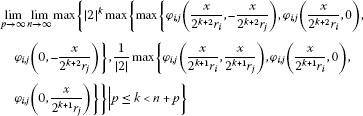then EL is the unique mapping satisfying (3.4).

Proof By (2.17), we know

$\begin{array}{rcl}\parallel f\left(x\right)-2f\left(\frac{x}{2}\right)\parallel & \le & max\left\{max\left\{{\phi }_{i,j}\left(\frac{x}{4{r}_{i}},-\frac{x}{4{r}_{j}}\right),{\phi }_{i,j}\left(\frac{x}{4{r}_{i}},0\right),{\phi }_{i,j}\left(0,-\frac{x}{4{r}_{j}}\right)\right\},\\ \frac{1}{|2|}max\left\{{\phi }_{i,j}\left(\frac{x}{2{r}_{i}},\frac{x}{2{r}_{j}}\right),{\phi }_{i,j}\left(\frac{x}{2{r}_{i}},0\right),{\phi }_{i,j}\left(0,\frac{x}{2{r}_{j}}\right)\right\}\right\}\end{array}$
(3.5)

for all $x\in G$. Replacing x by $\frac{x}{{2}^{n}}$ in (3.5), we obtain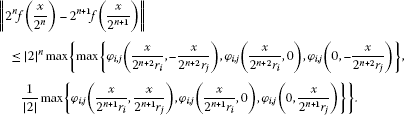(3.6)

It follows from (3.1) and (3.6) that the sequence ${\left\{{2}^{n}f\left(\frac{x}{{2}^{n}}\right)\right\}}_{n\ge 1}$ is a Cauchy sequence. Since X is complete, so ${\left\{{2}^{n}f\left(\frac{x}{{2}^{n}}\right)\right\}}_{n\ge 1}$ is convergent. Set

$\mathit{EL}\left(x\right):=\underset{n\to \mathrm{\infty }}{lim}{2}^{n}f\left(\frac{x}{{2}^{n}}\right).$

Using induction on n, one can show that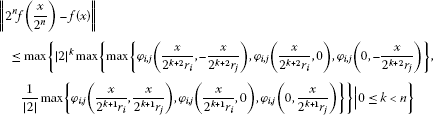(3.7)

for all $n\in \mathbb{N}$ and all $x\in G$. By taking n to approach infinity in (3.7) and using (3.2), one obtains (3.4). By (3.1) and (3.3), we get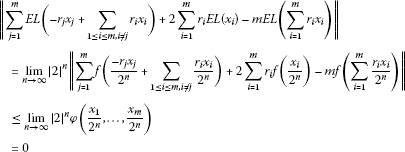for all ${x}_{1},\dots ,{x}_{m}\in X$. Therefore the function $\mathit{EL}:G\to X$ satisfies (1.1).

To prove the uniqueness property of EL, let $A:G\to X$ be another function satisfying (3.4). Then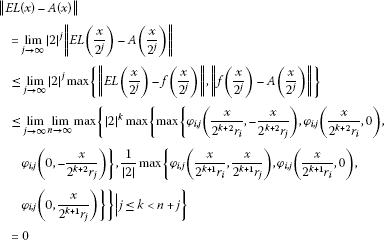for all $x\in G$. Therefore $A=\mathit{EL}$, and the proof is complete. □

Corollary 3.1 Let $\xi :\left[0,\mathrm{\infty }\right)\to \left[0,\mathrm{\infty }\right)$ be a function satisfying

$\xi \left(\frac{t}{|2|}\right)\le \xi \left(\frac{1}{|2|}\right)\xi \left(t\right)\phantom{\rule{1em}{0ex}}\left(t\ge 0\right)\phantom{\rule{2em}{0ex}}\xi \left(\frac{1}{|2|}\right)<{|2|}^{-1}.$
(3.8)

Let$\kappa >0$and$f:G\to X$be a mapping with$f\left(0\right)=0$satisfying the following inequality: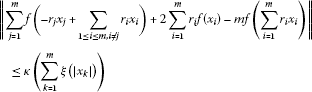(3.9)

for all${x}_{1},\dots ,{x}_{m}\in G$. Then there exists a unique Euler-Lagrange type additive mapping$\mathit{EL}:G\to X$such that

$\parallel f\left(x\right)-\mathit{EL}\left(x\right)\parallel \le \frac{\kappa }{|4|}\left\{\xi \left(|\frac{x}{{r}_{i}}|\right)+\xi \left(|\frac{x}{{r}_{j}}|\right)\right\}.$
(3.10)

Proof Defining $\zeta :{G}^{m}\to \left[0,\mathrm{\infty }\right)$ by $\phi \left({x}_{1},\dots ,{x}_{m}\right):=\kappa \left({\sum }_{k=1}^{m}\xi \left(|{x}_{k}|\right)\right)$, then we have

$\underset{n\to \mathrm{\infty }}{lim}{|2|}^{n}\phi \left(\frac{{x}_{1}}{{2}^{n}},\dots ,\frac{{x}_{m}}{{2}^{n}}\right)\le \underset{n\to \mathrm{\infty }}{lim}{\left(|2|\xi \left(\frac{1}{|2|}\right)\right)}^{n}\phi \left({x}_{1},\dots ,{x}_{m}\right)=0$

for all ${x}_{1},\dots ,{x}_{m}\in G$. On the other hand,

$\begin{array}{rcl}\mathrm{\Theta }\left(x\right)& =& \underset{n\to \mathrm{\infty }}{lim}max\left\{{|2|}^{k}max\left\{max\left\{{\phi }_{i,j}\left(\frac{x}{{2}^{k+2}{r}_{i}},-\frac{x}{{2}^{k+2}{r}_{j}}\right),{\phi }_{i,j}\left(\frac{x}{{2}^{k+2}{r}_{i}},0\right),\\ {\phi }_{i,j}\left(0,-\frac{x}{{2}^{k+2}{r}_{j}}\right)\right\},\frac{1}{|2|}max\left\{{\phi }_{i,j}\left(\frac{x}{{2}^{k+1}{r}_{i}},\frac{x}{{2}^{n+1}{r}_{j}}\right),{\phi }_{i,j}\left(\frac{x}{{2}^{k+1}{r}_{i}},0\right),\\ {\phi }_{i,j}\left(0,\frac{x}{{2}^{k+1}{r}_{j}}\right)\right\}\right\}|0\le k

for all $x\in G$, exists. Also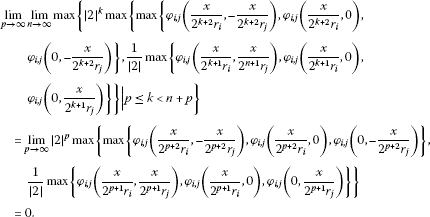Applying Theorem 3.1, we get the desired result. □

Theorem 3.2 Let $\phi :{G}^{m}\to \left[0,+\mathrm{\infty }\right)$ be a function such that

$\underset{n\to \mathrm{\infty }}{lim}\frac{\phi \left({2}^{n}{x}_{1},\dots ,{2}^{n}{x}_{m}\right)}{{|2|}^{n}}=0$
(3.11)

for all ${x}_{1},\dots ,{x}_{m}\in G$ and let for each $x\in G$ the limit

$\begin{array}{rcl}\mathrm{\Theta }\left(x\right)& =& \underset{n\to \mathrm{\infty }}{lim}max\left\{\frac{1}{{|2|}^{k}}max\left\{max\left\{{\phi }_{i,j}\left(\frac{{2}^{k-1}x}{{r}_{i}},-\frac{{2}^{k-1}x}{{r}_{j}}\right),\\ {\phi }_{i,j}\left(\frac{{2}^{k-1}x}{{r}_{i}},0\right),{\phi }_{i,j}\left(0,-\frac{{2}^{k-1}x}{{r}_{j}}\right)\right\},\\ \frac{1}{|2|}max\left\{{\phi }_{i,j}\left(\frac{{2}^{k}x}{{r}_{i}},\frac{{2}^{k}x}{{r}_{j}}\right),{\phi }_{i,j}\left(\frac{{2}^{k}x}{{r}_{i}},0\right),{\phi }_{i,j}\left(0,\frac{{2}^{k}x}{{r}_{j}}\right)\right\}\right\}|0\le k
(3.12)

exist. Suppose that$f:G\to X$is a mapping with$f\left(0\right)=0$satisfying (3.3). Then, the limit$\mathit{EL}\left(x\right):={lim}_{n\to \mathrm{\infty }}\frac{f\left({2}^{n}x\right)}{{2}^{n}}$exists for all$x\in G$and defines an Euler-Lagrange type additive mapping$\mathit{EL}:G\to X$, such that

$\parallel f\left(x\right)-\mathit{EL}\left(x\right)\parallel \le \frac{1}{|2|}\mathrm{\Theta }\left(x\right).$
(3.13)

Moreover, if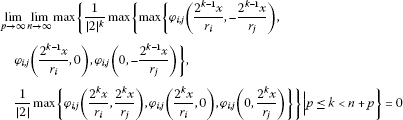then EL is the unique Euler-Lagrange type additive mapping satisfying (3.13).

Proof It follows from (2.16) that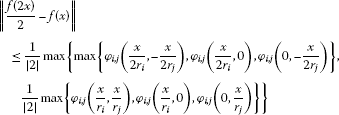(3.14)

for all $x\in G$. Replacing x by ${2}^{n}x$ in (3.14), we obtain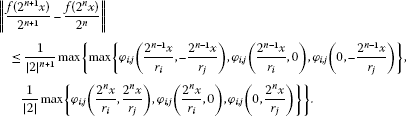(3.15)

It follows from (3.11) and (3.15) that the sequence ${\left\{\frac{f\left({2}^{n}x\right)}{{2}^{n}}\right\}}_{n\ge 1}$ is convergent. Set

$\mathit{EL}\left(x\right):=\underset{n\to \mathrm{\infty }}{lim}\frac{f\left({2}^{n}x\right)}{{2}^{n}}.$

On the other hand, it follows from (3.15) that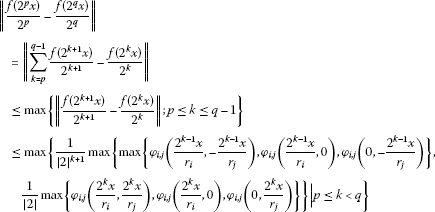for all $x\in G$ and all nonnegative integers p, q with $q>p\ge 0$. Letting $p=0$ and passing the limit $q\to \mathrm{\infty }$ in the last inequality and using (3.12), we obtain (3.13). The rest of the proof is similar to the proof of Theorem 3.1. □

Corollary 3.2 Let $\xi :\left[0,\mathrm{\infty }\right)\to \left[0,\mathrm{\infty }\right)$ be a function satisfying

$\xi \left(|2t|\right)\le \xi \left(|2|\right)\xi \left(t\right)\phantom{\rule{1em}{0ex}}\left(t\ge 0\right)\phantom{\rule{2em}{0ex}}\xi \left(|2|\right)<|2|.$
(3.16)

Let$\kappa >0$and$f:G\to X$be a mapping with$f\left(0\right)=0$satisfying the following inequality (3.9). Then there exists a unique Euler-Lagrange type additive mapping$\mathit{EL}:G\to X$such that

$\begin{array}{rcl}\parallel f\left(x\right)-\mathit{EL}\left(x\right)\parallel & \le & \frac{\kappa }{|2|}max\left\{\xi \left(|\frac{x}{2{r}_{i}}|\right)+\xi \left(|\frac{x}{2{r}_{j}}|\right),\frac{1}{|2|}\xi \left(|\frac{x}{{r}_{i}}|\right)+\xi \left(|\frac{x}{{r}_{j}}|\right)\right\}\\ =& \frac{\kappa }{|2|}\left[\xi \left(|\frac{x}{2{r}_{i}}|\right)+\xi \left(|\frac{x}{2{r}_{j}}|\right)\right].\end{array}$
(3.17)

Proof Defining $\zeta :{G}^{m}\to \left[0,\mathrm{\infty }\right)$ by $\phi \left({x}_{1},\dots ,{x}_{m}\right):=\kappa \left({\sum }_{k=1}^{m}\xi \left(|{x}_{k}|\right)\right)$, then, we have

$\underset{n\to \mathrm{\infty }}{lim}\frac{\phi \left({2}^{n}{x}_{1},\dots ,{2}^{n}{x}_{m}\right)}{{|2|}^{n}}\le \underset{n\to \mathrm{\infty }}{lim}{\left(\frac{\xi \left(|2|\right)}{|2|}\right)}^{n}\phi \left({x}_{1},\dots ,{x}_{m}\right)=0$

for all ${x}_{1},\dots ,{x}_{m}\in G$. On the other hand,

$\begin{array}{rcl}\mathrm{\Theta }\left(x\right)& =& \underset{n\to \mathrm{\infty }}{lim}max\left\{\frac{1}{{|2|}^{k}}max\left\{max\left\{{\phi }_{i,j}\left(\frac{{2}^{k-1}x}{{r}_{i}},-\frac{{2}^{k-1}x}{{r}_{j}}\right),\\ {\phi }_{i,j}\left(\frac{{2}^{k-1}x}{{r}_{i}},0\right),{\phi }_{i,j}\left(0,-\frac{{2}^{k-1}x}{{r}_{j}}\right)\right\},\\ \frac{1}{|2|}max\left\{{\phi }_{i,j}\left(\frac{{2}^{k}x}{{r}_{i}},\frac{{2}^{k}x}{{r}_{j}}\right),{\phi }_{i,j}\left(\frac{{2}^{k}x}{{r}_{i}},0\right),{\phi }_{i,j}\left(0,\frac{{2}^{k}x}{{r}_{j}}\right)\right\}\right\}|0\le k

for all $x\in G$, exists. Also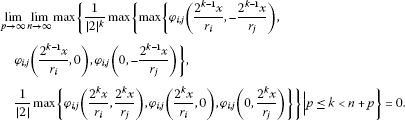Applying Theorem 3.14, we get the desired result. □

Remark 3.1 We remark that if $\xi \left(|2|\right)=0$, then $\xi =0$ identically, and so f is itself additive. Thus, for the nontrivial ξ, we observe that $\xi \left(|2|\right)\ne 0$ and

$1\le \xi \left(|1|\right)\le \xi \left(|2|\right)\xi \left(\frac{1}{|2|}\right)\le |2|\xi \left(\frac{1}{|2|}\right)$

implies that $\frac{1}{|2|}\le \xi \left(\frac{1}{|2|}\right)$.

## References

1. Arriola LM, Beyer WA: Stability of the Cauchy functional equation over p -adic fields. Real Anal. Exch. 2005/06, 31: 125–132.

2. Balcerowski M:On the functional equation $f\left(x+g\left(y\right)\right)-f\left(y+g\left(y\right)\right)=f\left(x\right)-f\left(y\right)$ on groups. Aequ. Math. 2009, 78: 247–255. 10.1007/s00010-009-2975-9

3. Cho YJ, Park C, Saadati R: Functional inequalities in non-Archimedean in Banach spaces. Appl. Math. Lett. 2010, 60: 1994–2002.

4. Cho YJ, Saadati R: Lattice non-Archimedean random stability of ACQ functional equation. Adv. Differ. Equ. 2011., 2011:

5. Cholewa PW: Remarks on the stability of functional equations. Aequ. Math. 1984, 27: 76–86. 10.1007/BF02192660

6. Czerwik S: Functional Equations and Inequalities in Several Variables. World Scientific, River Edge; 2002.

7. Ebadian A, Ghobadipour N, Gordji ME:A fixed point method for perturbation of bimultipliers and Jordan bimultipliers in ${C}^{\ast }$-ternary algebras. J. Math. Phys. 2010., 51(10):

8. Ebadian A, Kaboli Gharetapeh S, Eshaghi Gordji M: Nearly Jordan -homomorphisms between unital ${C}^{\ast }$ -algebras. Abstr. Appl. Anal. 2011., 2011:

9. Ebadian A, Najati A, Eshaghi Gordji M: On approximate additive-quartic and quadratic-cubic functional equations in two variables on abelian groups. Results Math. 2010, 58(1–2):39–53. 10.1007/s00025-010-0018-4

10. Eshaghi Gordji M: Nearly ring homomorphisms and nearly ring derivations on non-Archimedean Banach algebras. Abstr. Appl. Anal. 2010., 2010:

11. Eshaghi Gordji M: Stability of a functional equation deriving from quartic and additive functions. Bull. Korean Math. Soc. 2010, 47(3):491–502. 10.4134/BKMS.2010.47.3.491

12. Eshaghi Gordji M: Stability of an additive-quadratic functional equation of two variables in F -spaces. J. Nonlinear Sci. Appl. 2009, 2(4):251–259.

13. Eshaghi Gordji M, Abbaszadeh S, Park C: On the stability of generalized mixed type quadratic and quartic functional equation in quasi-Banach spaces. J. Inequal. Appl. 2009., 2009:

14. Eshaghi Gordji M, Alizadeh Z: Stability and superstability of ring homomorphisms on non-Archimedean Banach algebras. Abstr. Appl. Anal. 2011., 2011:

15. Gǎvruta P: A generalization of the Hyers-Ulam-Rassias stability of approximately additive mappings. J. Math. Anal. Appl. 1994, 184: 431–436. 10.1006/jmaa.1994.1211

16. Gselmann E, Maksa G: Stability of the parametric fundamental equation of information for nonpositive parameters. Aequ. Math. 2009, 78: 271–282. 10.1007/s00010-009-2980-z

17. Hyers DH: On the stability of the linear functional equation. Proc. Natl. Acad. Sci. USA 1941, 27: 222–224. 10.1073/pnas.27.4.222

18. Mihet D, Radu V: On the stability of the additive Cauchy functional equation in random normed spaces. J. Math. Anal. Appl. 2008, 343: 567–572. 10.1016/j.jmaa.2008.01.100

19. Najati A, Kang JI, Cho YJ: Local stability of the pexiderized Cauchy and Jensen’s equations in fuzzy spaces. J. Inequal. Appl. 2011., 2011:

20. Najati A, Cho YJ: Generalized Hyers-Ulam stability of the pexiderized Cauchy functional equation in non-Archimedean spaces. Fixed Point Theory Appl. 2011., 2011:

21. Najati A, Park C:Stability of a generalized Euler-Lagrange type additive mapping and homomorphisms in ${C}^{\ast }$-algebras. J. Nonlinear Sci. Appl. 2010, 3(2):134–154.

22. Park C: Fuzzy stability of a functional equation associated with inner product spaces. Fuzzy Sets Syst. 2009, 160: 1632–1642. 10.1016/j.fss.2008.11.027

23. Park C: Generalized Hyers-Ulam-Rassias stability of n -sesquilinear-quadratic mappings on Banach modules over ${C}^{\ast }$ -algebras. J. Comput. Appl. Math. 2005, 180: 279–291. 10.1016/j.cam.2004.11.001

24. Rassias TM: On the stability of the linear mapping in Banach spaces. Proc. Am. Math. Soc. 1978, 72: 297–300. 10.1090/S0002-9939-1978-0507327-1

25. Rassias TM: On the stability of the quadratic functional equation and its applications. Stud. Univ. Babeş-Bolyai, Math. 1998, XLIII: 89–124.

26. Rassias TM: The problem of S. M. Ulam for approximately multiplicative mappings. J. Math. Anal. Appl. 2000, 246: 352–378. 10.1006/jmaa.2000.6788

27. Rassias TM: On the stability of functional equations in Banach spaces. J. Math. Anal. Appl. 2000, 251: 264–284. 10.1006/jmaa.2000.7046

28. Rassias TM: On the stability of functional equations and a problem of Ulam. Acta Appl. Math. 2000, 62: 23–130. 10.1023/A:1006499223572

29. Rassias TM, Šemrl P: On the Hyers-Ulam stability of linear mappings. J. Math. Anal. Appl. 1993, 173: 325–338. 10.1006/jmaa.1993.1070

30. Rassias TM, Shibata K: Variational problem of some quadratic functionals in complex analysis. J. Math. Anal. Appl. 1998, 228: 234–253. 10.1006/jmaa.1998.6129

31. Saadati R, Cho YJ, Vahidi J: The stability of the quartic functional equation in various spaces. Comput. Math. Appl. 2010, 60: 1994–2002. 10.1016/j.camwa.2010.07.034

32. Saadati R, Vaezpour M, Cho YJ: A note to paper ‘On the stability of cubic mappings and quartic mappings in random normed spaces’. J. Inequal. Appl. 2009., 2009:

33. Saadati R, Zohdi MM, Vaezpour SM: Nonlinear L -random stability of an ACQ functional equation. J. Inequal. Appl. 2011., 2011:

34. Ulam SM: Problems in Modern Mathematics. Wiley, New York; 1940. Chapter VI

## Acknowledgement

Dong Yun Shin was supported by the 2011 sabbatical year research grant of the University of Seoul.

## Author information

Authors

### Corresponding author

Correspondence to DY Shin.

### Competing interests

The authors declare that they have no competing interests.

### Authors’ contributions

All authors conceived of the study participated in its design and coordination, drafted the manuscript, participated in the sequence alignment, and read and approved the final manuscript.

## Rights and permissions

Reprints and Permissions

Azadi Kenary, H., Rezaei, H., Sharifzadeh, M. et al. Non-Archimedean Hyers-Ulam-Rassias stability of m-variable functional equation. Adv Differ Equ 2012, 111 (2012). https://doi.org/10.1186/1687-1847-2012-111